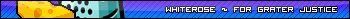The question about good AA algorithm for small scaling (float values not integer

Started by dullman, September 06, 2014, 09:11:53 pm

dullmanSeptember 06, 2014, 09:11:53 pm
Hello i'm not sure if i post in right part of forum, but since it rather general question although it will be used by rpg maker (to be precise dll that is used by rpg maker).
So my question i have a standard height of characters equals 170 cm = 105 px, so i want to my characters have a different height depends on their heights, (so if i have a character of height 150 it will be 150/170 * 105 px, also it will be used to enlarger characters height if their height is more then 170). So i checked the wiki for standard ones algorithm with AA to scale them but all algorithms are used with integer values, so i want to know if there is an description for algorithm that scales images in such small scale (and float factor), Or they might be two algorithms one to scale down one to scale up.

WhiteRoseSeptember 06, 2014, 09:33:31 pm #1
I think the problem with scaling at such a small level is that you can't have float values "in between" pixels. Pixels can only deal with whole numbers, so you can't have half a pixel be one thing and half be the other thing.

I might just be misunderstanding, but I hope that helps.winkioSeptember 06, 2014, 09:53:38 pm #2
As far as I know the most common set of algorithms are simple polynomial interpolations/extrapolations, with increasing order of equation: linear, quadratic, cubic, etc.  These equations should work independently of whether you use float or integer values.  Something to note is that higher order equations require more data points and work more slowly, so if you are doing this in-game you should probably settle on quadratic or cubic.

So yeah, google search quadratic interpolation or cubic interpolation, you should find the 1-dimensional equations pretty easily, but you will have to either search harder or do some work yourself to get the 2-dimensional equations.  Then just run them on the R, G, B, and A channels separately (or altogether in vector form) and you should be good to go.